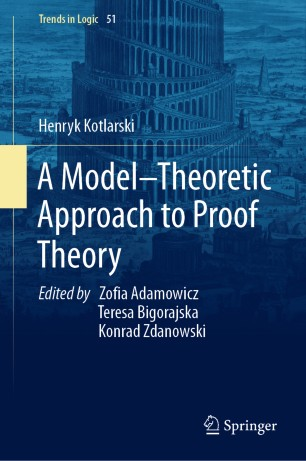# A Model–Theoretic Approach to Proof Theory

• Henryk Kotlarski
• Teresa BigorajskaBook

Part of the Trends in Logic book series (TREN, volume 51)

1. Front Matter
Pages i-xviii
2. Henryk Kotlarski
Pages 1-37
3. Henryk Kotlarski
Pages 39-42
4. Henryk Kotlarski
Pages 43-71
5. Henryk Kotlarski
Pages 73-87
6. Henryk Kotlarski
Pages 89-106
7. Back Matter
Pages 107-109

### Introduction

This book presents a detailed treatment of ordinal combinatorics of large sets tailored for independence results. It uses model theoretic and combinatorial methods to obtain results in proof theory, such as incompleteness theorems or a description of the provably total functions of a theory.

In the first chapter, the authors first discusses ordinal combinatorics of finite sets in the style of Ketonen and Solovay. This provides a background for an analysis of subsystems of Peano Arithmetic as well as for combinatorial independence results. Next, the volume examines a variety of proofs of Gödel's incompleteness theorems. The presented proofs differ strongly in nature. They show various aspects of incompleteness phenomena. In additon, coverage introduces some classical methods like the arithmetized completeness theorem, satisfaction predicates or partial satisfaction classes. It also applies them in many contexts.

The fourth chapter defines the method of indicators for obtaining independence results. It shows what amount of transfinite induction we have in fragments of Peano arithmetic. Then, it uses combinatorics of large sets of the first chapter to show independence results. The last chapter considers nonstandard satisfaction classes. It presents some of the classical theorems related to them. In particular, it covers the results by S. Smith on definability in the language with a satisfaction class and on models without a satisfaction class.

Overall, the book's content lies on the border between combinatorics, proof theory, and model theory of arithmetic. It offers readers a distinctive approach towards independence results by model-theoretic methods.

### Keywords

Gödel's Incompleteness Theorems Model Theory of Arithmetic Nonstandard Satisfaction Classes Proofs of Incompleteness Theorems Combinatorics of Alfa Large Sets Ketonen-Solovay Largeness Notion Independence Results for Peano Arithmetic Arithmetized Completeness Theorem Transfinite Induction in Arithmetic Hardy Hierarchy of Functions model theoretic ordinal combinatorics subsystems of Peano Arithmetic

#### Authors and affiliations

• Henryk Kotlarski
• 1
1. 1.(deceased)WarsawPoland